前言：

Python POC编写 的第一篇 ，

什么是EXP、POC、CVE：

POCProof Of Concept，观点验证程序。

EXPexploit（漏洞利用程序），就是利用代码

CVECommon Vulnerabilities & Exposures 公共漏洞和暴露。

输入输出：

Python语法比较简单，这里就不做更多说明了。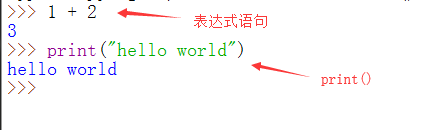数据类型：

Python3中有6个标准数据类型：

1. Number(数字)
2. String(字符串)
3. List(列表)
4. Tuple(元组)
5. Sets(集合)
6. Dictionary(字典)

1. Number(数字)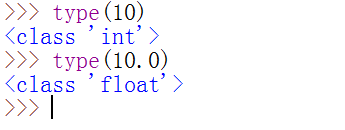2. String(字符串)

"hello world"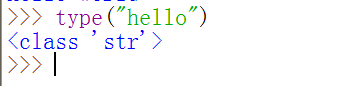3. List(列表)

list2 = [1,2,3,"小明",10.1,[1,2,3]]

1. append() 函数是将参数作为一个元素增加到列表的末尾。
2. extend() 方法则是将参数作为一个列表去扩展列表的末尾。

list2.append(1)  # 向列表list2末尾增加一个整数
list2.append("hello")  # 向列表list2末尾增加一个字符串
list2.append([1,2,3])  # 向列表list2末尾增加一个列表

list2.extend([1,2,3])

append()extend() 都是向列表末尾增加元素，我们来看看两者的区别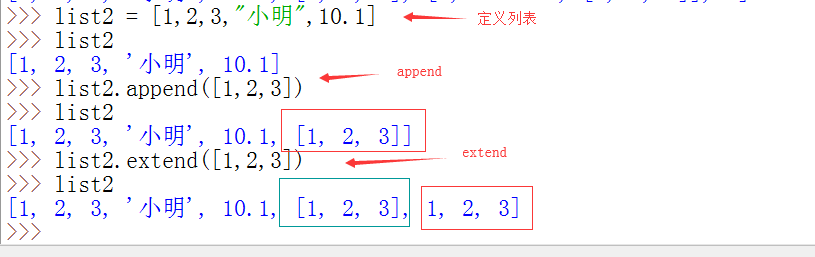list2

list2[1:5]

list2[6:]

list2[:6]

list2[:-2]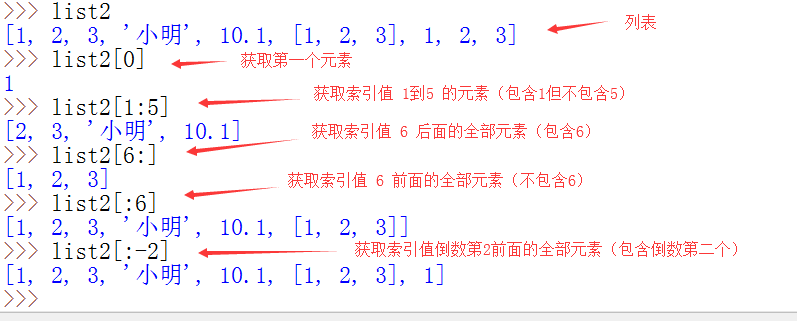del list2  # 删除第一个元素

list2[2:5] = []  # 指定为空

del list2

list2 = []

list2 = 233   # 将第一个元素的值改变为 整数233，如果使用单引号或者双引号就是字符串

4. Tuple(元组)

tup1 = (1,2,3)

tup1 = 1,2,3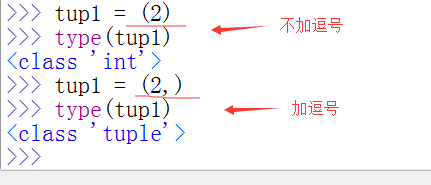tup1 = (1,2,3,4,5,6)
tup1 = tup1[:2] + ("小明",) + tup1[2:]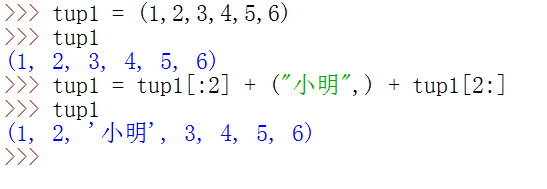5. Sets(集合)

a = set('abcdefggfedcba')

b = {1,2,3,4,5,5,4,3,2,1}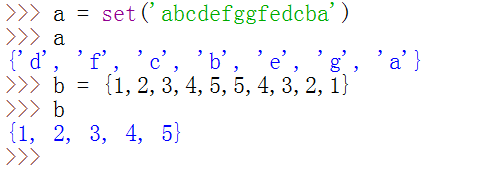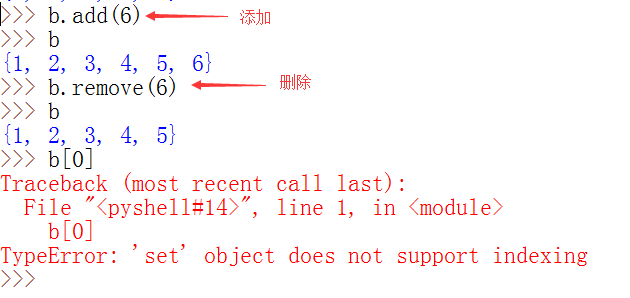6. Dictionary(字典)

dict1 = { 'a':123,'b':456,'7':789 }

dict2 = dict((('a',123),('b',456),('7',789)))

dict1['a']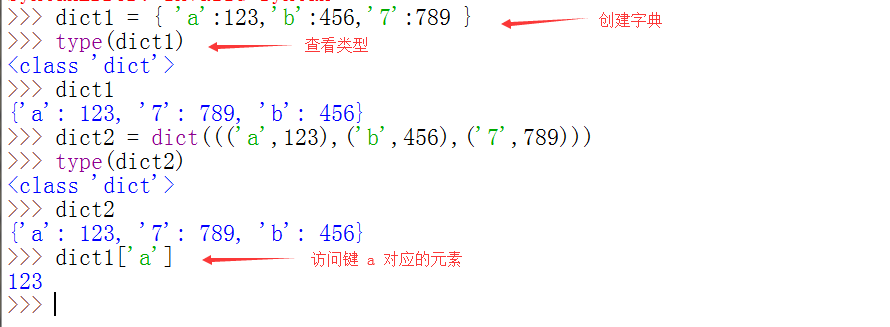流程控制：

if语句：

if 1 < 2:
print('窝喜欢')

if 1 < 2:
print('窝喜欢你')
else:
print('窝还是喜欢你')

if 1 < 2:
print('窝喜欢')
elif 2 < 1:
print('窝还是喜欢你')
else:
print('窝真喜欢你')

if 1 < 2:
print('窝喜欢')
if 2 < 1:
print('窝还是喜欢你')
else:
print('窝真喜欢你')

while语句：

while 条件:
循环体

count = 0
while count <= 10 :
print("loop ", count)
if count == 5:
break
count +=1

break：用于结束一个循环

count5 的时候，结束此次循环。

count = 0
while count <= 10 :
print("loop ", count)
if count == 5:
continue
count +=1

continue：只是终止本次循环，后面会接着继续循环。

while else 语句：

count = 0
while count <= 5 :
print("loop ", count)
count +=1
else:
print("循环结束啦！！！");

else 作用是指，当 while 循环正常执行完，中间没有被 break 中止的话，就会执行else后面的语句，